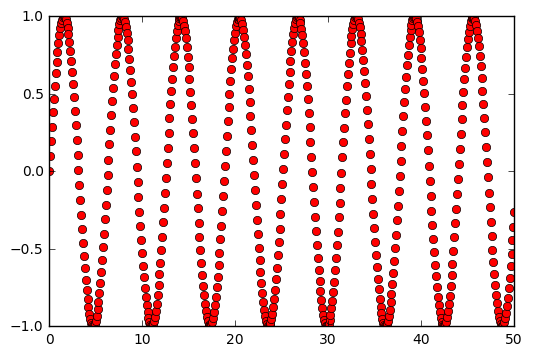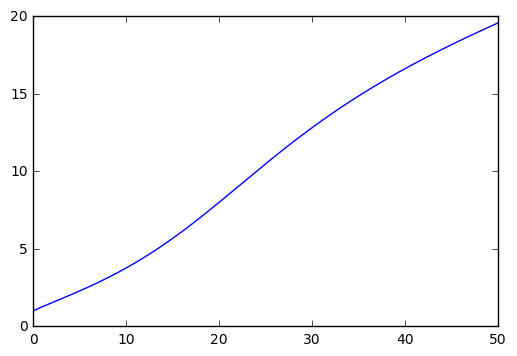# Solving differential equations numerically¶

Analytic functions are certainly very nice ... but sometimes you just want to know the answer, eh?

References:

Jim Mahoney | Jan 2018

In :
# I'm using python 2.
# Install some numerical and plotting packages. ("numpy" is "numerical python")
from numpy import *
from matplotlib.pyplot import plot
import matplotlib.pyplot as plt
import numpy as np
% matplotlib inline

In :
# Define t as a list of 100 numbers from 0.0 to 10.0.

t = np.linspace(0, 50, num=512)

print "Here are the first few values of t :"
print t[0:10]

# Then to see for example sin(t), we can just take the sin of each of those.
# (There is an implied loop here - we're finding [sin(t), sin(t), ...]

print "And here are the first few values of sin(t) :"
print sin(t)[0:10]

print "And here's a picture ..."

plot( t, sin(t) , 'ro')
plt.show()

Here are the first few values of t :
[ 0.          0.09784736  0.19569472  0.29354207  0.39138943  0.48923679
0.58708415  0.68493151  0.78277886  0.88062622]
And here are the first few values of sin(t) :
[ 0.          0.0976913   0.19444804  0.2893446   0.38147315  0.46995235
0.55393576  0.63261997  0.70525223  0.77113773]
And here's a picture ...We can use these arrays of numbers to solve differentiatl equations.

Well, difference equations actually, but for small $\Delta t$ it's nearly the same. :)

As an example, let's solve

$$\frac{dx}{dt} = \frac{1}{3+sin(\sqrt{t}))} = f(t)$$

where x=1 at t=0. (I'm just making this up - it's an example, eh?)

We put t on a grid , something like

$$t = [ 0.01, 0.02, 0.03, ... ]$$

and approximate the derivative with a difference equation

$$\frac{dx}{dt} = \frac{x[n+1] - x[n]}{\Delta t}$$

This lets us solve for x[n+1] in terms of t and x[n] :

$$x[n+1] = x[n] + (\Delta t) f(t)$$
In :
# Here we go.

x = zeros(len(t))

In :
x

Out:
array([ 0.,  0.,  0.,  0.,  0.,  0.,  0.,  0.,  0.,  0.,  0.,  0.,  0.,
0.,  0.,  0.,  0.,  0.,  0.,  0.,  0.,  0.,  0.,  0.,  0.,  0.,
0.,  0.,  0.,  0.,  0.,  0.,  0.,  0.,  0.,  0.,  0.,  0.,  0.,
0.,  0.,  0.,  0.,  0.,  0.,  0.,  0.,  0.,  0.,  0.,  0.,  0.,
0.,  0.,  0.,  0.,  0.,  0.,  0.,  0.,  0.,  0.,  0.,  0.,  0.,
0.,  0.,  0.,  0.,  0.,  0.,  0.,  0.,  0.,  0.,  0.,  0.,  0.,
0.,  0.,  0.,  0.,  0.,  0.,  0.,  0.,  0.,  0.,  0.,  0.,  0.,
0.,  0.,  0.,  0.,  0.,  0.,  0.,  0.,  0.,  0.,  0.,  0.,  0.,
0.,  0.,  0.,  0.,  0.,  0.,  0.,  0.,  0.,  0.,  0.,  0.,  0.,
0.,  0.,  0.,  0.,  0.,  0.,  0.,  0.,  0.,  0.,  0.,  0.,  0.,
0.,  0.,  0.,  0.,  0.,  0.,  0.,  0.,  0.,  0.,  0.,  0.,  0.,
0.,  0.,  0.,  0.,  0.,  0.,  0.,  0.,  0.,  0.,  0.,  0.,  0.,
0.,  0.,  0.,  0.,  0.,  0.,  0.,  0.,  0.,  0.,  0.,  0.,  0.,
0.,  0.,  0.,  0.,  0.,  0.,  0.,  0.,  0.,  0.,  0.,  0.,  0.,
0.,  0.,  0.,  0.,  0.,  0.,  0.,  0.,  0.,  0.,  0.,  0.,  0.,
0.,  0.,  0.,  0.,  0.,  0.,  0.,  0.,  0.,  0.,  0.,  0.,  0.,
0.,  0.,  0.,  0.,  0.,  0.,  0.,  0.,  0.,  0.,  0.,  0.,  0.,
0.,  0.,  0.,  0.,  0.,  0.,  0.,  0.,  0.,  0.,  0.,  0.,  0.,
0.,  0.,  0.,  0.,  0.,  0.,  0.,  0.,  0.,  0.,  0.,  0.,  0.,
0.,  0.,  0.,  0.,  0.,  0.,  0.,  0.,  0.,  0.,  0.,  0.,  0.,
0.,  0.,  0.,  0.,  0.,  0.,  0.,  0.,  0.,  0.,  0.,  0.,  0.,
0.,  0.,  0.,  0.,  0.,  0.,  0.,  0.,  0.,  0.,  0.,  0.,  0.,
0.,  0.,  0.,  0.,  0.,  0.,  0.,  0.,  0.,  0.,  0.,  0.,  0.,
0.,  0.,  0.,  0.,  0.,  0.,  0.,  0.,  0.,  0.,  0.,  0.,  0.,
0.,  0.,  0.,  0.,  0.,  0.,  0.,  0.,  0.,  0.,  0.,  0.,  0.,
0.,  0.,  0.,  0.,  0.,  0.,  0.,  0.,  0.,  0.,  0.,  0.,  0.,
0.,  0.,  0.,  0.,  0.,  0.,  0.,  0.,  0.,  0.,  0.,  0.,  0.,
0.,  0.,  0.,  0.,  0.,  0.,  0.,  0.,  0.,  0.,  0.,  0.,  0.,
0.,  0.,  0.,  0.,  0.,  0.,  0.,  0.,  0.,  0.,  0.,  0.,  0.,
0.,  0.,  0.,  0.,  0.,  0.,  0.,  0.,  0.,  0.,  0.,  0.,  0.,
0.,  0.,  0.,  0.,  0.,  0.,  0.,  0.,  0.,  0.,  0.,  0.,  0.,
0.,  0.,  0.,  0.,  0.,  0.,  0.,  0.,  0.,  0.,  0.,  0.,  0.,
0.,  0.,  0.,  0.,  0.,  0.,  0.,  0.,  0.,  0.,  0.,  0.,  0.,
0.,  0.,  0.,  0.,  0.,  0.,  0.,  0.,  0.,  0.,  0.,  0.,  0.,
0.,  0.,  0.,  0.,  0.,  0.,  0.,  0.,  0.,  0.,  0.,  0.,  0.,
0.,  0.,  0.,  0.,  0.,  0.,  0.,  0.,  0.,  0.,  0.,  0.,  0.,
0.,  0.,  0.,  0.,  0.,  0.,  0.,  0.,  0.,  0.,  0.,  0.,  0.,
0.,  0.,  0.,  0.,  0.,  0.,  0.,  0.,  0.,  0.,  0.,  0.,  0.,
0.,  0.,  0.,  0.,  0.,  0.,  0.,  0.,  0.,  0.,  0.,  0.,  0.,
0.,  0.,  0.,  0.,  0.])
In :
dt = t - t
dt

Out:
0.097847358121330719
In :
def f(t):
return 1.0/(3.0 + sin(sqrt(t)))

In :
f(0.1234)

Out:
0.29903384442636777
In :
# Start here :
x = 1.0

# And now use the difference equation to get the next value ... over and over.
for i in range(1, len(t)):
_t = (i - 0.5) * dt   # midway between x[i-1] and x[i]
x[i] = x[i-1] + dt * f(_t)

plot(t, x)
plt.show()In :
# All right, not a very exciting plot ... but that's the idea.

# The point is that you can do this sort of thing to get
# numerical solutions to equations that can't be done analytically
# ... which is most of 'em.


TODO

• more examples
• 2nd order equations
• some physics equations : forced damped harmonic oscillator, schrodinder equation, ...
• nondimensionalization (i.e. "get rid of the physical units")
In [ ]: